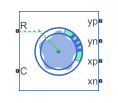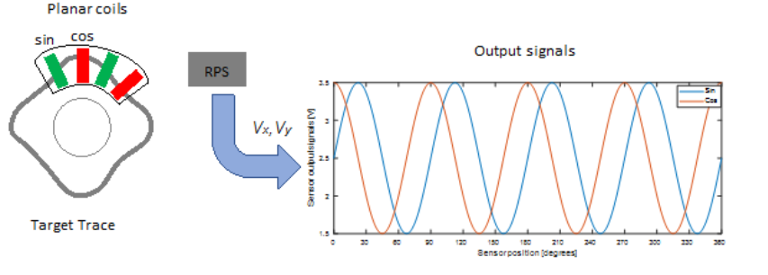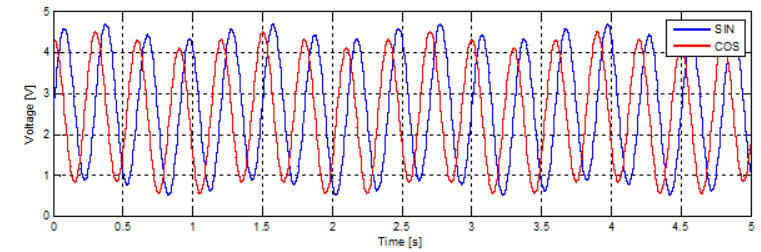# Inductive Rotor Position Sensor

Inductive rotor position sensor with four inductive coils

• Library:
• Simscape / Electrical / Sensors & Transducers

•## Description

The Inductive Rotor Position Sensor block uses the theory of eddy current losses to obtain the rotor position. It consists of four planar coils and a target trace that detect the rotor position. The target trace is shaped in a sinusoidal way and made from conductive material. The distance between the four planar coils is equivalent to 90 degrees of one cycle.### Equations

The voltages between the elements of the sensor are described by the equations:

`${v}_{x}={V}_{x0}+{A}_{x}\mathrm{cos}\left(N\theta \right)+K\mathrm{sin}\left(\theta \right)$`

`${v}_{y}={V}_{y0}+{A}_{y}\mathrm{cos}\left(N\theta -\frac{\pi }{2}+\beta \right)+K\mathrm{sin}\left(\theta \right)$`

where:

• vx is the cosine voltage.

• vy is the sine voltage.

• Ax and Ay are the voltage amplitudes for the x and y axes that reflect the sensitivity mismatch.

• Vx0 and Vy0 are the voltage offsets for the x and y axes.

• N is the number of pole pairs.

• θ is the mechanical position.

• β is the quadrature error.

• K is the tumbling factor.

The block uses this equation to decode the angle:

`${\theta }_{out}=\mathrm{arctan}\left(\frac{{v}_{y}}{{v}_{x}}\right).$`

This figure shows the effects of the tumbling factor:### Variables

To set the priority and initial target values for the block variables prior to simulation, use the Initial Targets section in the block dialog box or Property Inspector. For more information, see Set Priority and Initial Target for Block Variables.

Nominal values provide a way to specify the expected magnitude of a variable in a model. Using system scaling based on nominal values increases the simulation robustness. Nominal values can come from different sources, one of which is the Nominal Values section in the block dialog box or Property Inspector. For more information, see System Scaling by Nominal Values.

## Ports

### Conserving

expand all

Mechanical rotational conserving port associated with the sensor positive probe.

Mechanical rotational conserving port associated with the sensor negative (reference) probe.

Electrical conserving port associated with the `y`-axis positive terminal.

#### Dependencies

To enable this port, set Output interface to `Electrical connections`.

Electrical conserving port associated with the `y`-axis negative terminal.

#### Dependencies

To enable this port, set Output interface to `Electrical connections`.

Electrical conserving port associated with the `x`-axis positive terminal.

#### Dependencies

To enable this port, set Output interface to `Electrical connections`.

Electrical conserving port associated with the `x`-axis negative terminal.

#### Dependencies

To enable this port, set Output interface to `Electrical connections`.

### Output

expand all

Rotational angle of the magnetic field in the x-y plane, returned as a physical signal.

#### Dependencies

To enable this port, set Output interface to `Decoded angular position`.

## Parameters

expand all

Number of pole pairs.

`x`-axis voltage amplitude.

`y`-axis voltage amplitude.

Potential difference in the electric current flow through the electric conductor in the absence of external magnetic field on the `x`-axis.

Potential difference in the electric current flow through the electric conductor in the absence of external magnetic field on the `y`-axis.

Amount of quadrature error. Quadrature switching can decrease offset errors.

Tumbling coefficient, in degrees.

Port interface for the decoded information, specified as either ```Electrical connections``` or ```Decoded angular position```.

## Version History

Introduced in R2020a